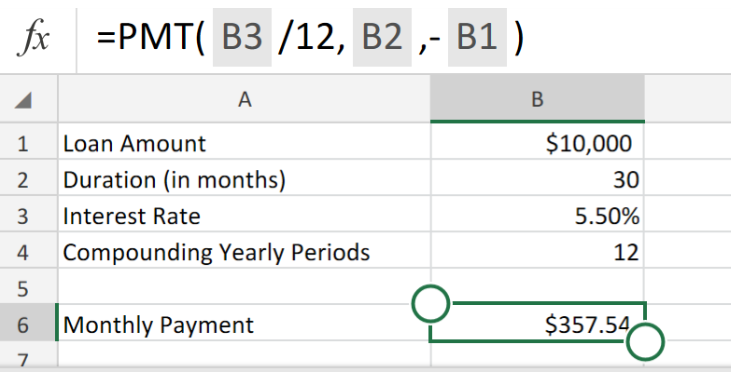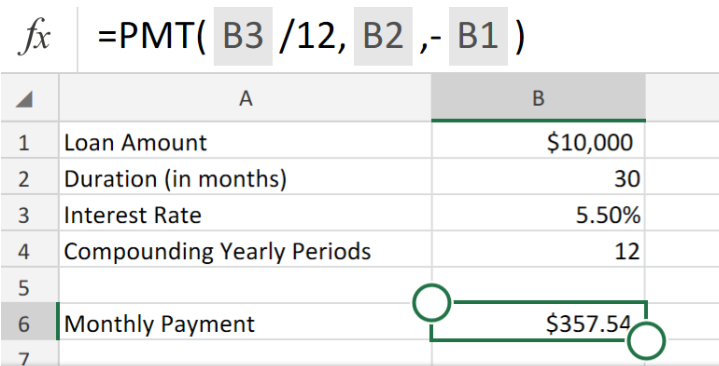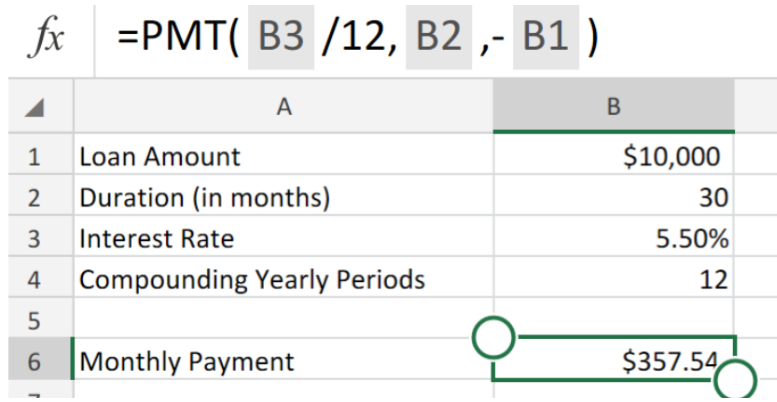Get instant live expert help with Excel or Google Sheets“My Excelchat expert helped me in less than 20 minutes, saving me what would have been 5 hours of work!”

#### Post your problem and you'll get expert help in seconds

Your message must be at least 40 characters
Our professional experts are available now. Your privacy is guaranteed.

# The Interest Rate Function in ExcelFigure 1. of Interest Rate Calculation in Excel.

The Interest Rate Function in Excel allows us to calculate per period of a loan.

In this post, we are going to walk through the usage and formula syntax of the Rate Function in Excel.

## Generic Formula

`=PMT(rate,periods,-amount)`

The components of the operation syntax for the PMT Function are as follows;

• nper – the number of monthly durations/periods.
• rate – Interest Rate per duration.
• pv – the initial loan amount.

## How to use Calculate the Interest Rate for a Loan in Excel.

To determine the amount due for loan payment, given a loan duration/term, a Rate of Interest, and the initial loan amount, we can utilize the Excel PMT Operation Syntax..

In the example illustrated below, the operation syntax inserted into the formula bar of cell B6 is thus-

`=PMT (B3/12,B2,-B1)`

This calculates the monthly payment with interest for the loan.Figure 2. of Excel PMT Function.

Loans consist of 4 basic parts. The Loan amount, Rate of Interest, the loan duration (number of regular payments), and an amount to be paid per period. We can use the Excel PMT Function to calculate the payment amount when we have all four components.Figure 3. of Final Result.

## Instant Connection to an Expert through our Excelchat Service:

Our live Excelchat Service is here for you. We have Excel Experts available 24/7 to answer any Excel questions you may have. Guaranteed connection within 30 seconds and a customized solution for you within 20 minutes

### Did this post not answer your question? Get a solution from connecting with the expert.Another blog reader asked this question today on Excelchat:## Subscribe to Excelchat.coAnother blog reader asked this question today on Excelchat: